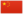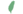—— 燕麦掌对影族的评论，雷影交加》，第15章

Oatclaw燕麦爪 Oatpaw  燕麥掌
 燕麦掌 Oatclaw  燕麥爪

Q3359：风族公猫，自《黑莓星的风暴》登场的角色

## 细节

### 外貌

• 他是一只浅棕色虎斑公猫。:猫物表

## 语录

—— 狮焰和燕麦掌，关于一星还剩多少条命，《雷影交加》，第66页

—— 花楸星和燕麦掌，关于暗尾猫群，《雷影交加》，第181页

## 登场书目

• 学徒探索 (Q170)[猫物闲角]
• 雷影交加 (Q171)[配角]
• 天空破碎 (Q172)[配角]
• 极夜无光 (Q173)[猫物闲角]
• 烈焰焚河 (Q174)[猫物闲角]
• 风暴来袭 (Q175)[猫物闲角]
• 迷失群星 (Q3563)[猫物闲角]
• 静默冰融 (Q3579)[猫物闲角]
• 叠影重障 (Q3624)[猫物闲角]
• 暗由心生 (Q4183)[猫物闲角]
• 无星之地 (Q4385)[猫物闲角]
• 迷雾明光 (Q4621)[猫物闲角]
• 河翻浪涌 (Q4643)[配角]
• 黑莓星的风暴 (Q182)[猫物闲角][首次登场🐱]
• 虎心的阴影 (Q185)[猫物闲角]
• 鸦羽的拷问 (Q186)[配角]
• 松鼠飞的希冀 (Q3561)[配角]
• 灰条的誓言 (Q4057)[猫物闲角]
• 鸽翅的沉默 (Q192)[猫物闲角]
• 褐皮的族群 (Q3566)[猫物闲角]
• 黛西的至亲 (Q3629)[猫物闲角]
• 斑毛的叛变 (Q4337)[猫物闲角]
• ## 参考文献

这篇文章基于CC BY-SA 3.0许可使用了猫武士维基（英语）Oatclaw一文中的部分内容。
0.0
0人评价